# RD Sharma Solutions Class 12 Linear Programming Exercise 30.3

RD Sharma Solutions for Class 12 Maths Chapter 30 Linear Programming Exercise 30.3, is provided here for students to prepare and score good marks in the exams. Our experts have solved the difficult problems into simpler steps which can be easily solved by students. Regular revision of important concepts and formulas over time is the best way to strengthen your concepts. Students are advised to practice the RD Sharma Class 12 Solutions regularly to achieve their desired scores. The pdf consisting of this chapter solutions which are available for free can be downloaded from the links given below.

## Download PDF of RD Sharma Solutions for Class 12 Maths Chapter 30 Exercise 3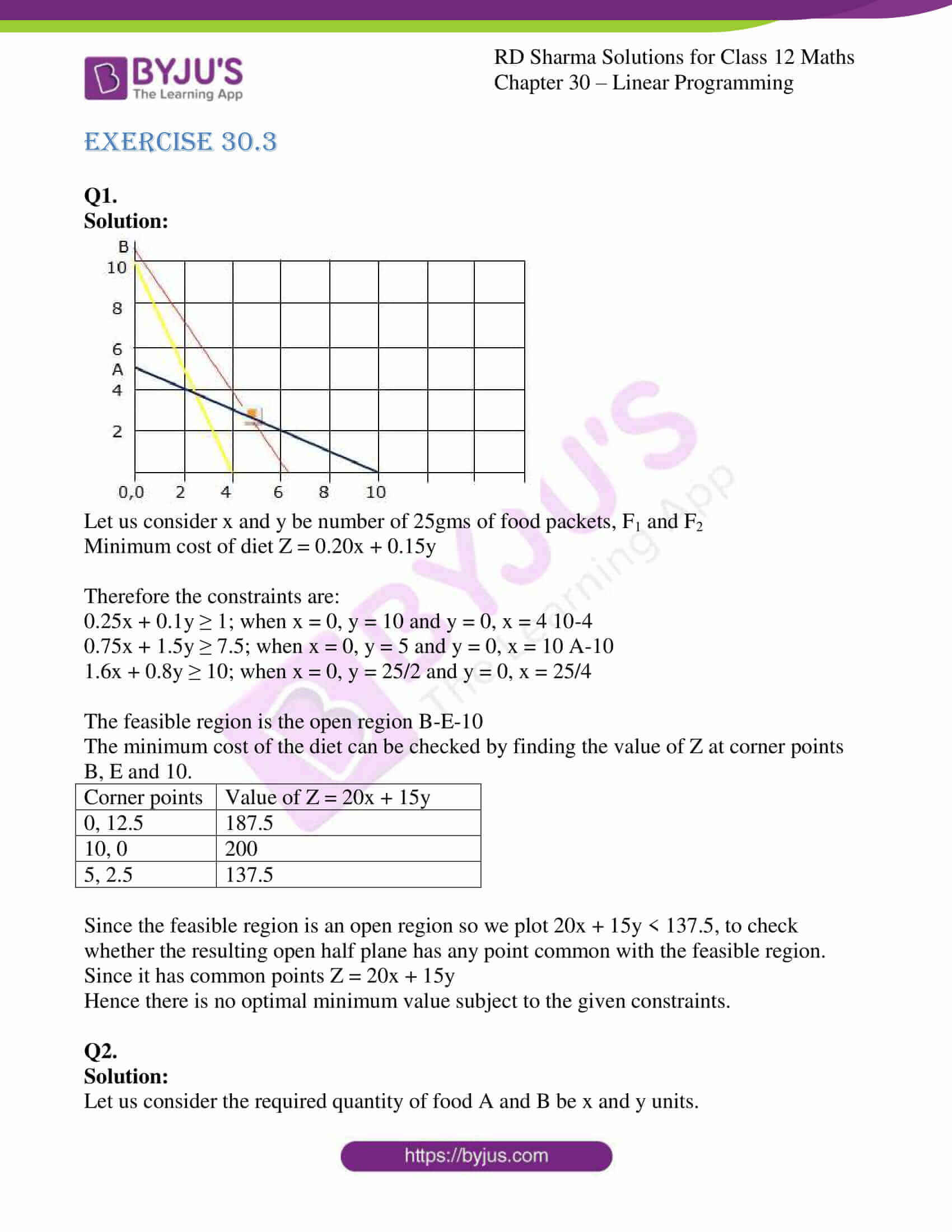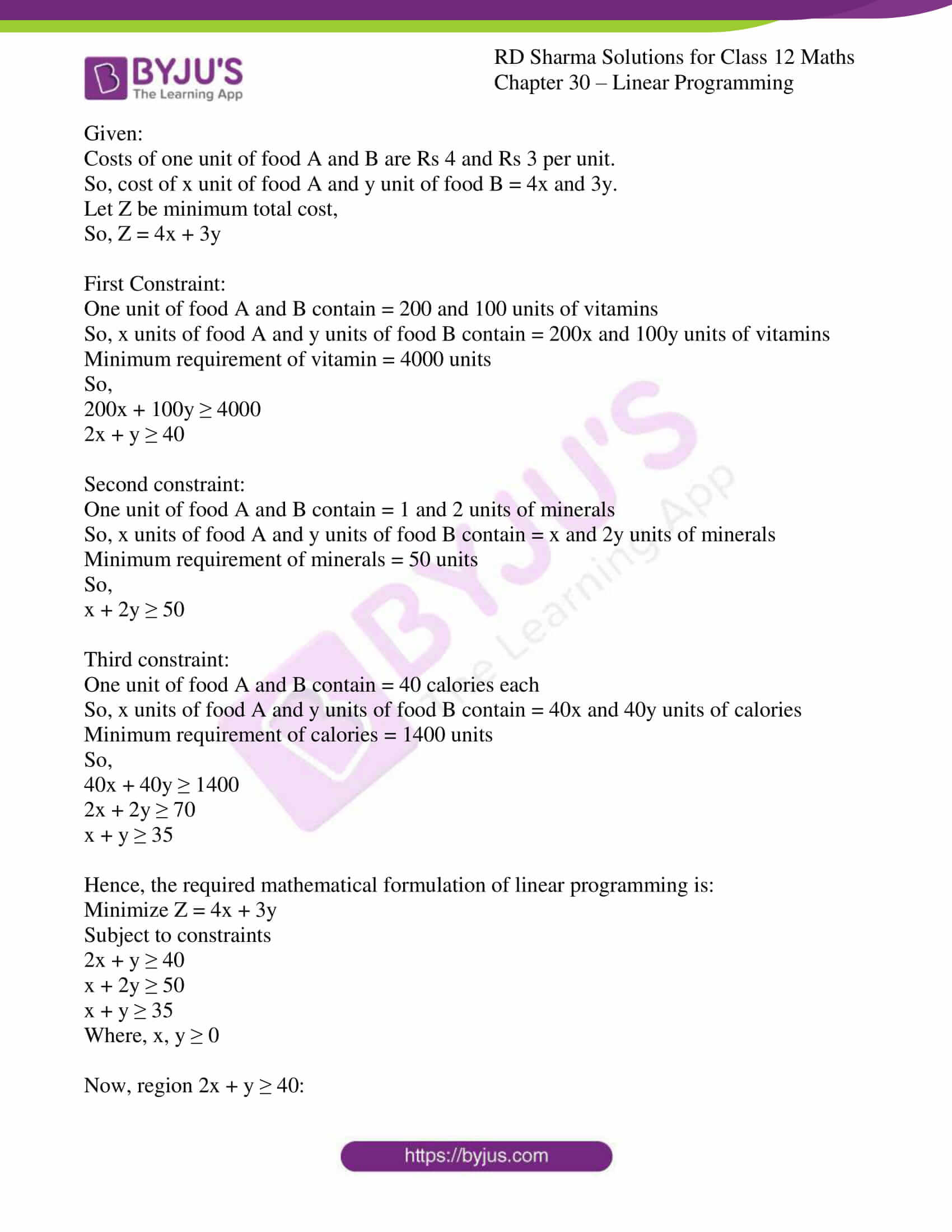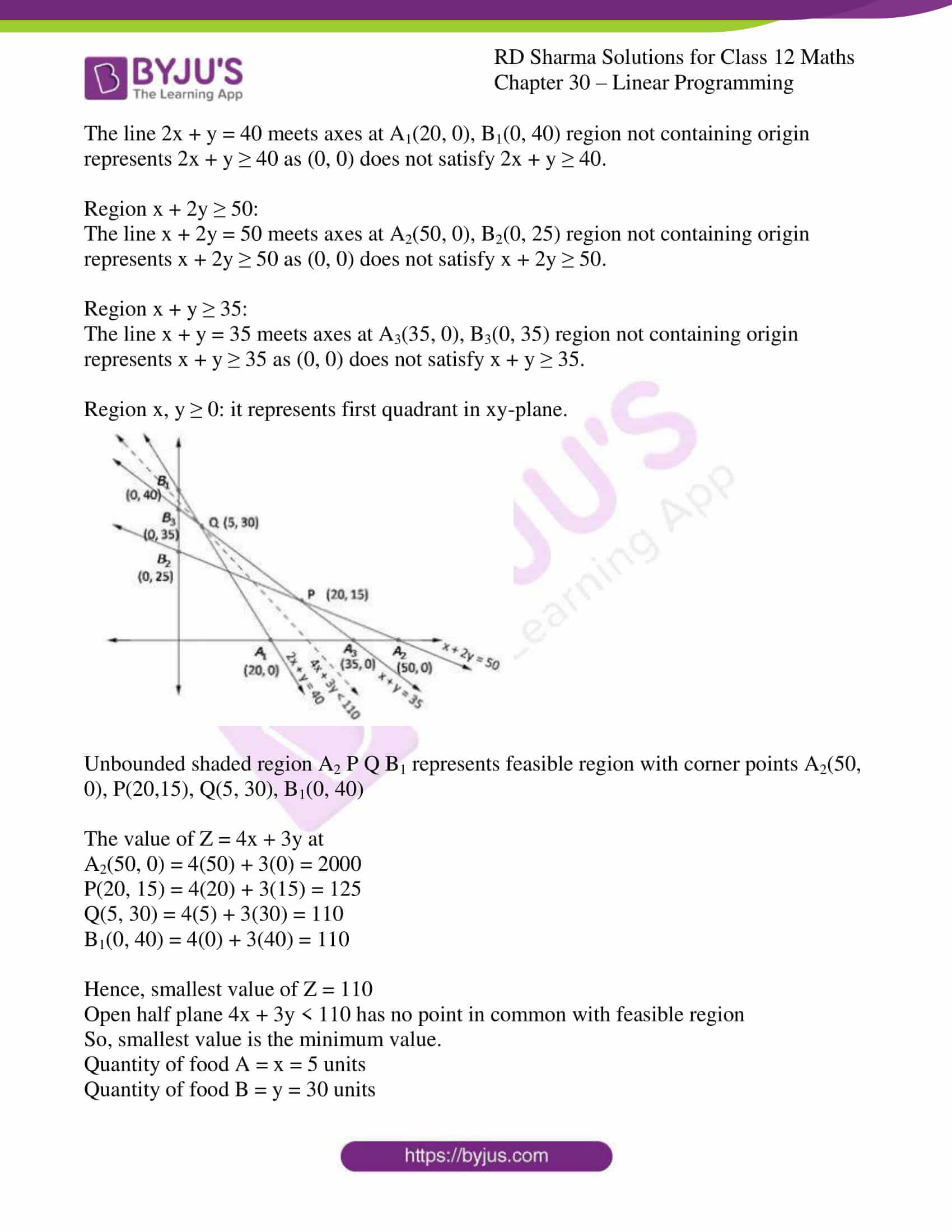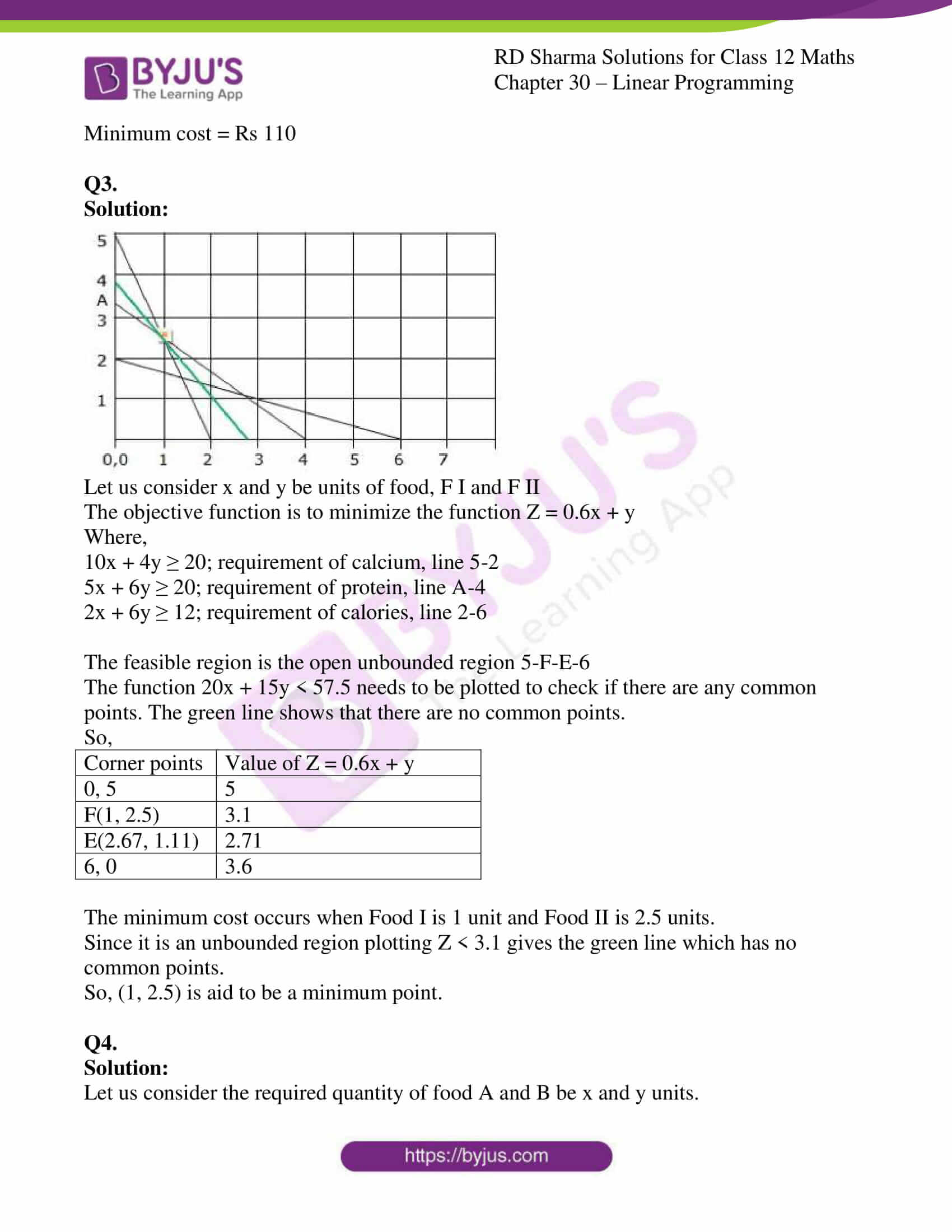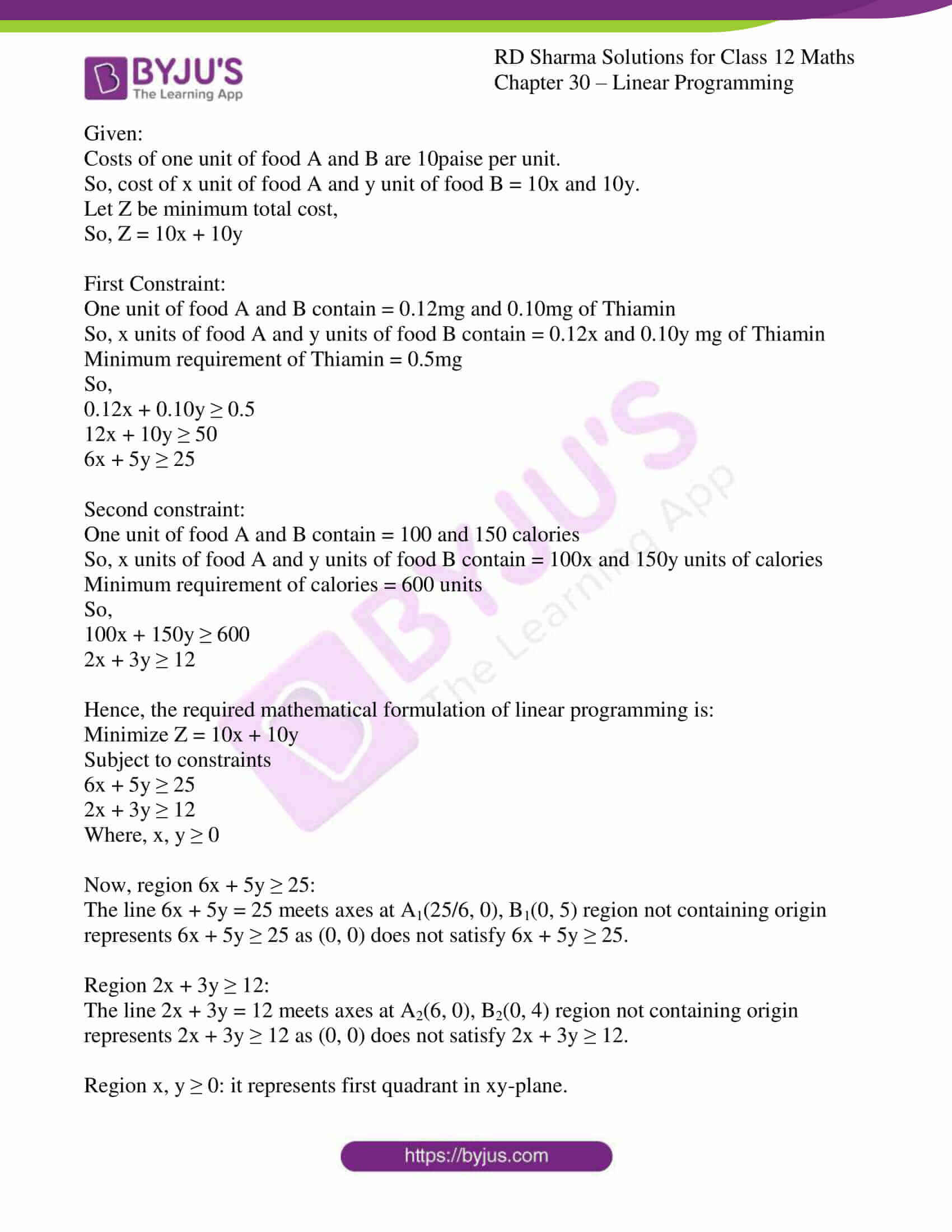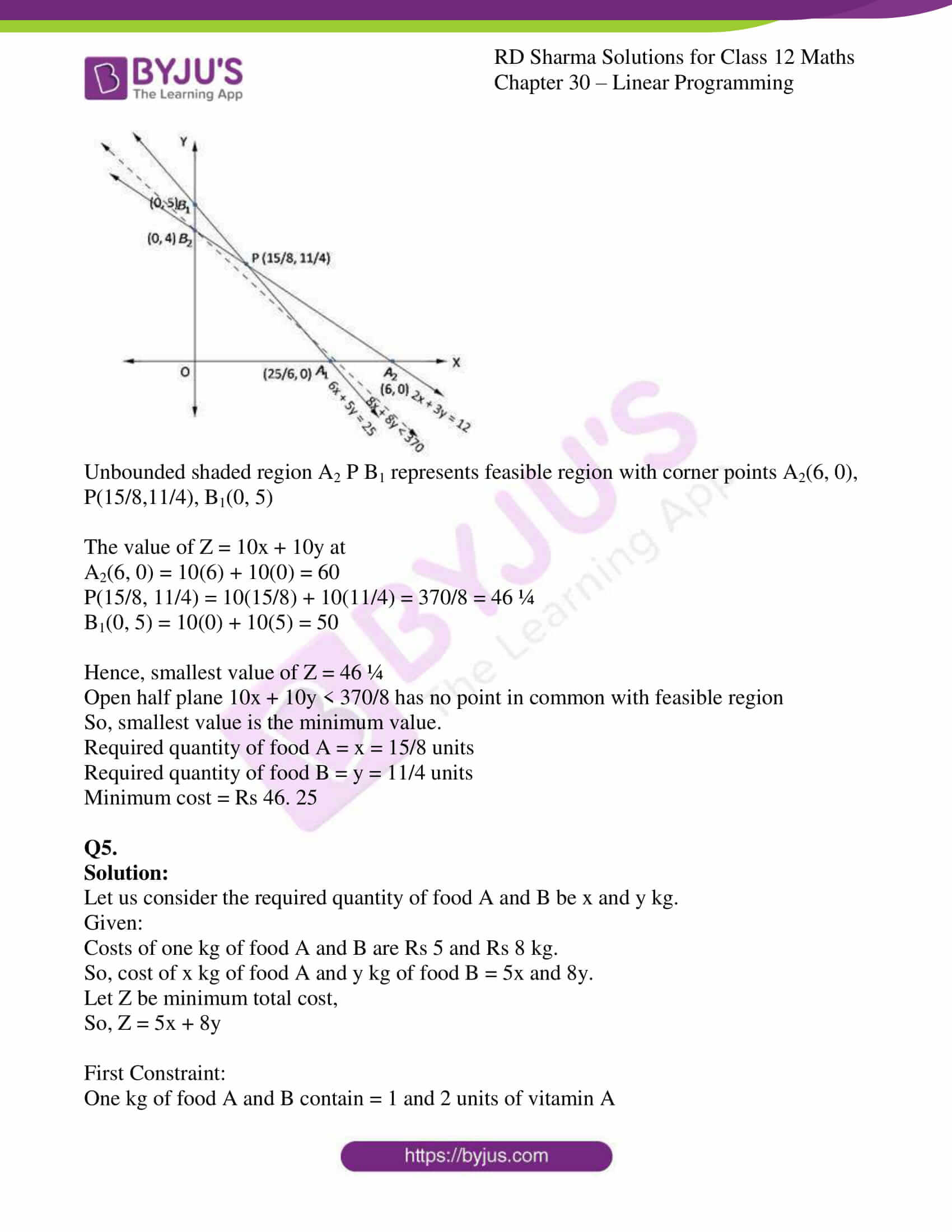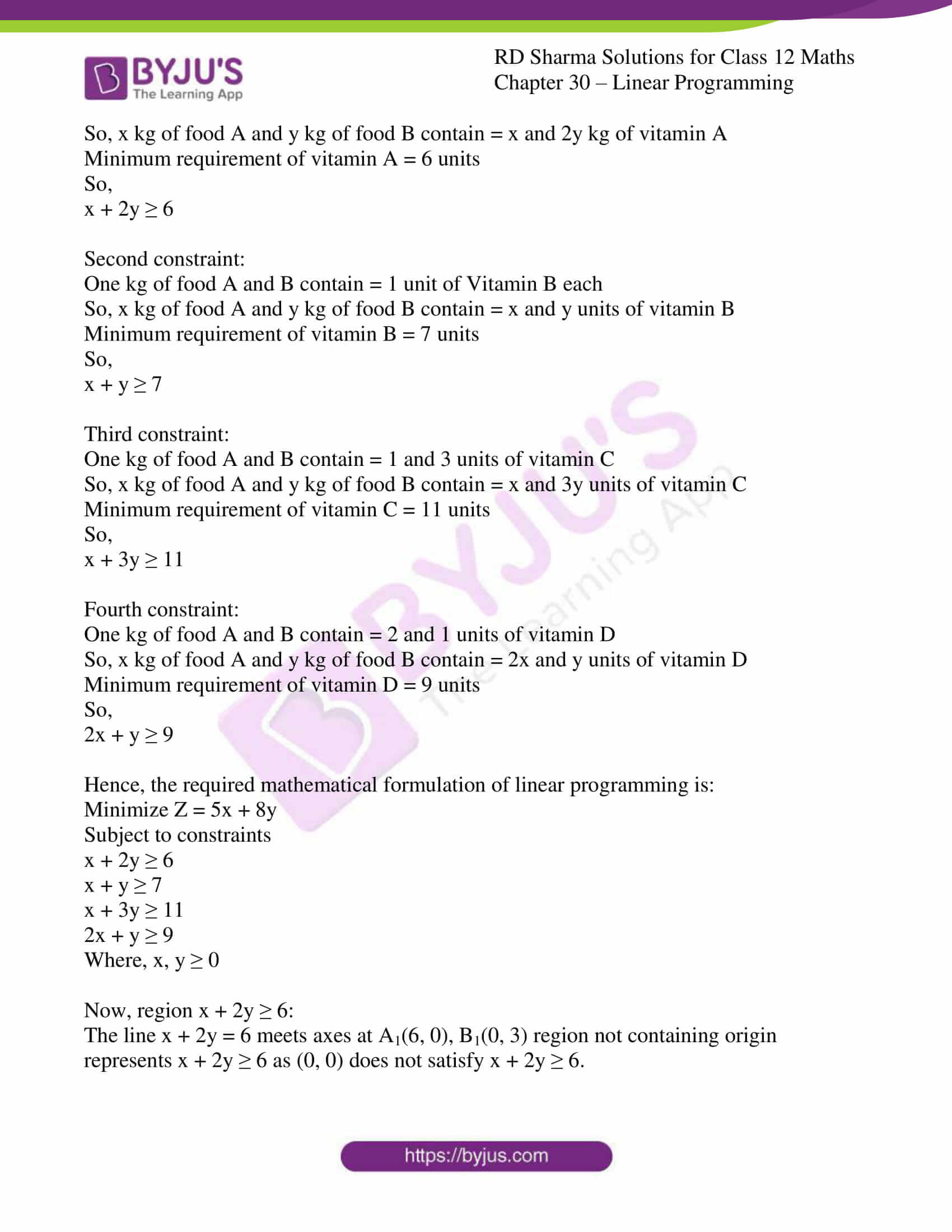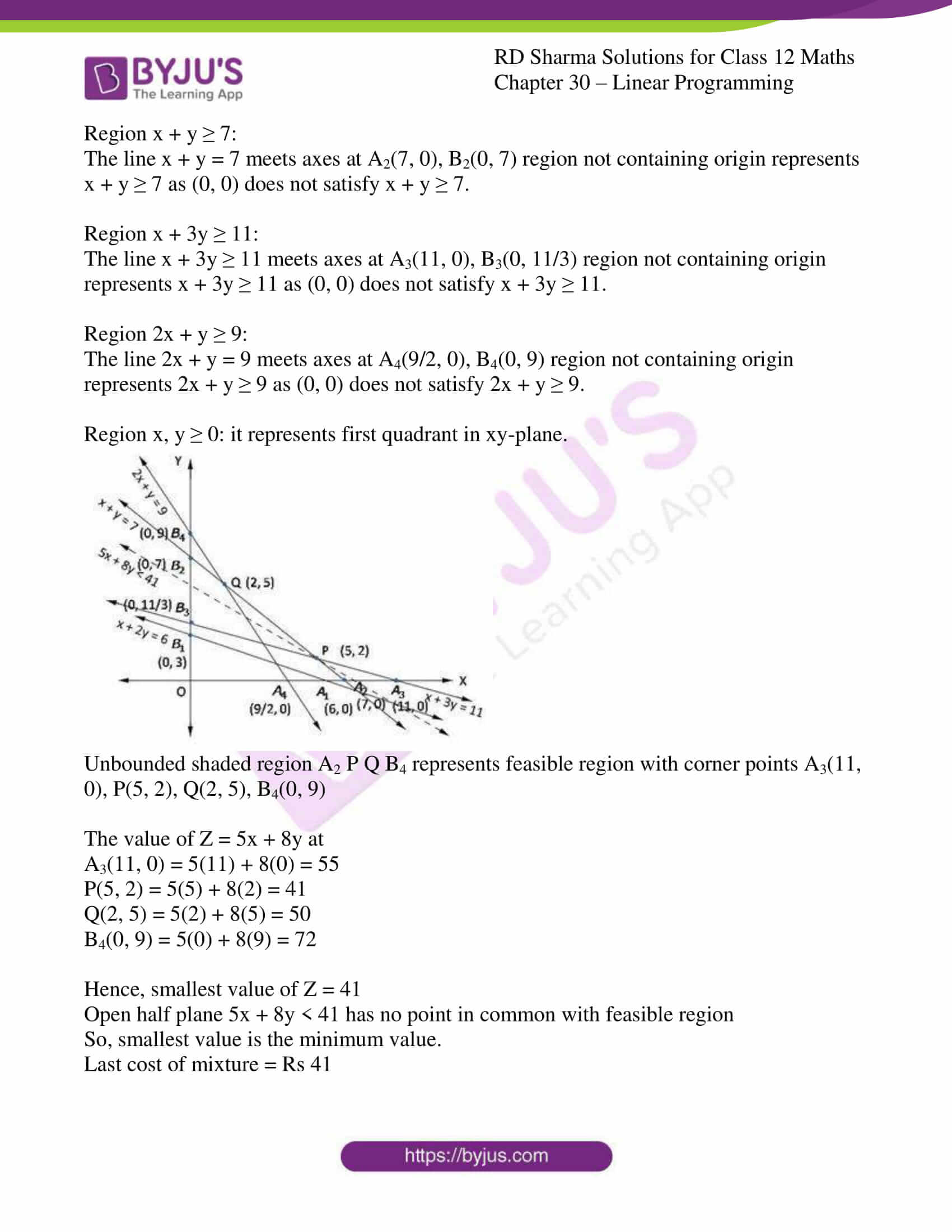### Access RD Sharma Solutions for Class 12 Maths Chapter 30 Exercise 3

EXERCISE 30.3

Q1.

Solution: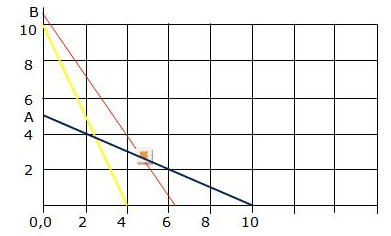Let us consider x and y be number of 25gms of food packets, F1 and F2

Minimum cost of diet Z = 0.20x + 0.15y

Therefore the constraints are:

0.25x + 0.1y ≥ 1; when x = 0, y = 10 and y = 0, x = 4 10-4

0.75x + 1.5y ≥ 7.5; when x = 0, y = 5 and y = 0, x = 10 A-10

1.6x + 0.8y ≥ 10; when x = 0, y = 25/2 and y = 0, x = 25/4

The feasible region is the open region B-E-10

The minimum cost of the diet can be checked by finding the value of Z at corner points B, E and 10.

 Corner points Value of Z = 20x + 15y 0, 12.5 187.5 10, 0 200 5, 2.5 137.5

Since the feasible region is an open region so we plot 20x + 15y < 137.5, to check whether the resulting open half plane has any point common with the feasible region.

Since it has common points Z = 20x + 15y

Hence there is no optimal minimum value subject to the given constraints.

Q2.

Solution:

Let us consider the required quantity of food A and B be x and y units.

Given:

Costs of one unit of food A and B are Rs 4 and Rs 3 per unit.

So, cost of x unit of food A and y unit of food B = 4x and 3y.

Let Z be minimum total cost,

So, Z = 4x + 3y

First Constraint:

One unit of food A and B contain = 200 and 100 units of vitamins

So, x units of food A and y units of food B contain = 200x and 100y units of vitamins

Minimum requirement of vitamin = 4000 units

So,

200x + 100y ≥ 4000

2x + y ≥ 40

Second constraint:

One unit of food A and B contain = 1 and 2 units of minerals

So, x units of food A and y units of food B contain = x and 2y units of minerals

Minimum requirement of minerals = 50 units

So,

x + 2y ≥ 50

Third constraint:

One unit of food A and B contain = 40 calories each

So, x units of food A and y units of food B contain = 40x and 40y units of calories

Minimum requirement of calories = 1400 units

So,

40x + 40y ≥ 1400

2x + 2y ≥ 70

x + y ≥ 35

Hence, the required mathematical formulation of linear programming is:

Minimize Z = 4x + 3y

Subject to constraints

2x + y ≥ 40

x + 2y ≥ 50

x + y ≥ 35

Where, x, y ≥ 0

Now, region 2x + y ≥ 40:

The line 2x + y = 40 meets axes at A1(20, 0), B1(0, 40) region not containing origin represents 2x + y ≥ 40 as (0, 0) does not satisfy 2x + y ≥ 40.

Region x + 2y ≥ 50:

The line x + 2y = 50 meets axes at A2(50, 0), B2(0, 25) region not containing origin represents x + 2y ≥ 50 as (0, 0) does not satisfy x + 2y ≥ 50.

Region x + y ≥ 35:

The line x + y = 35 meets axes at A3(35, 0), B3(0, 35) region not containing origin represents x + y ≥ 35 as (0, 0) does not satisfy x + y ≥ 35.

Region x, y ≥ 0: it represents first quadrant in xy-plane.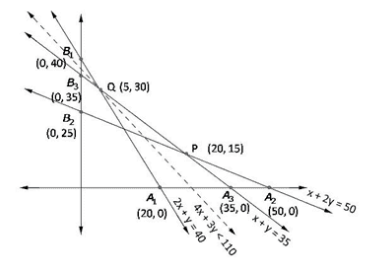Unbounded shaded region A2 P Q B1 represents feasible region with corner points A2(50, 0), P(20,15), Q(5, 30), B1(0, 40)

The value of Z = 4x + 3y at

A2(50, 0) = 4(50) + 3(0) = 2000

P(20, 15) = 4(20) + 3(15) = 125

Q(5, 30) = 4(5) + 3(30) = 110

B1(0, 40) = 4(0) + 3(40) = 110

Hence, smallest value of Z = 110

Open half plane 4x + 3y < 110 has no point in common with feasible region

So, smallest value is the minimum value.

Quantity of food A = x = 5 units

Quantity of food B = y = 30 units

Minimum cost = Rs 110

Q3.

Solution: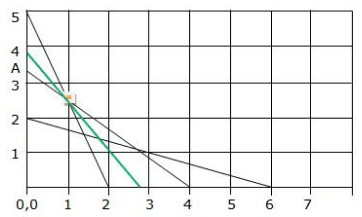Let us consider x and y be units of food, F I and F II

The objective function is to minimize the function Z = 0.6x + y

Where,

10x + 4y ≥ 20; requirement of calcium, line 5-2

5x + 6y ≥ 20; requirement of protein, line A-4

2x + 6y ≥ 12; requirement of calories, line 2-6

The feasible region is the open unbounded region 5-F-E-6

The function 20x + 15y < 57.5 needs to be plotted to check if there are any common points. The green line shows that there are no common points.

So,

 Corner points Value of Z = 0.6x + y 0, 5 5 F(1, 2.5) 3.1 E(2.67, 1.11) 2.71 6, 0 3.6

The minimum cost occurs when Food I is 1 unit and Food II is 2.5 units.

Since it is an unbounded region plotting Z < 3.1 gives the green line which has no common points.

So, (1, 2.5) is aid to be a minimum point.

Q4.

Solution:

Let us consider the required quantity of food A and B be x and y units.

Given:

Costs of one unit of food A and B are 10paise per unit.

So, cost of x unit of food A and y unit of food B = 10x and 10y.

Let Z be minimum total cost,

So, Z = 10x + 10y

First Constraint:

One unit of food A and B contain = 0.12mg and 0.10mg of Thiamin

So, x units of food A and y units of food B contain = 0.12x and 0.10y mg of Thiamin

Minimum requirement of Thiamin = 0.5mg

So,

0.12x + 0.10y ≥ 0.5

12x + 10y ≥ 50

6x + 5y ≥ 25

Second constraint:

One unit of food A and B contain = 100 and 150 calories

So, x units of food A and y units of food B contain = 100x and 150y units of calories

Minimum requirement of calories = 600 units

So,

100x + 150y ≥ 600

2x + 3y ≥ 12

Hence, the required mathematical formulation of linear programming is:

Minimize Z = 10x + 10y

Subject to constraints

6x + 5y ≥ 25

2x + 3y ≥ 12

Where, x, y ≥ 0

Now, region 6x + 5y ≥ 25:

The line 6x + 5y = 25 meets axes at A1(25/6, 0), B1(0, 5) region not containing origin represents 6x + 5y ≥ 25 as (0, 0) does not satisfy 6x + 5y ≥ 25.

Region 2x + 3y ≥ 12:

The line 2x + 3y = 12 meets axes at A2(6, 0), B2(0, 4) region not containing origin represents 2x + 3y ≥ 12 as (0, 0) does not satisfy 2x + 3y ≥ 12.

Region x, y ≥ 0: it represents first quadrant in xy-plane.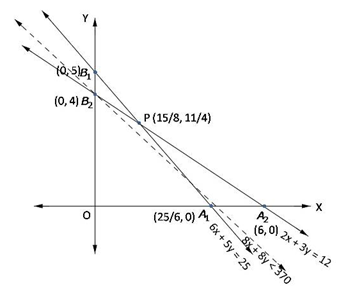Unbounded shaded region A2 P B1 represents feasible region with corner points A2(6, 0), P(15/8,11/4), B1(0, 5)

The value of Z = 10x + 10y at

A2(6, 0) = 10(6) + 10(0) = 60

P(15/8, 11/4) = 10(15/8) + 10(11/4) = 370/8 = 46 ¼

B1(0, 5) = 10(0) + 10(5) = 50

Hence, smallest value of Z = 46 ¼

Open half plane 10x + 10y < 370/8 has no point in common with feasible region

So, smallest value is the minimum value.

Required quantity of food A = x = 15/8 units

Required quantity of food B = y = 11/4 units

Minimum cost = Rs 46. 25

Q5.

Solution:

Let us consider the required quantity of food A and B be x and y kg.

Given:

Costs of one kg of food A and B are Rs 5 and Rs 8 kg.

So, cost of x kg of food A and y kg of food B = 5x and 8y.

Let Z be minimum total cost,

So, Z = 5x + 8y

First Constraint:

One kg of food A and B contain = 1 and 2 units of vitamin A

So, x kg of food A and y kg of food B contain = x and 2y kg of vitamin A

Minimum requirement of vitamin A = 6 units

So,

x + 2y ≥ 6

Second constraint:

One kg of food A and B contain = 1 unit of Vitamin B each

So, x kg of food A and y kg of food B contain = x and y units of vitamin B

Minimum requirement of vitamin B = 7 units

So,

x + y ≥ 7

Third constraint:

One kg of food A and B contain = 1 and 3 units of vitamin C

So, x kg of food A and y kg of food B contain = x and 3y units of vitamin C

Minimum requirement of vitamin C = 11 units

So,

x + 3y ≥ 11

Fourth constraint:

One kg of food A and B contain = 2 and 1 units of vitamin D

So, x kg of food A and y kg of food B contain = 2x and y units of vitamin D

Minimum requirement of vitamin D = 9 units

So,

2x + y ≥ 9

Hence, the required mathematical formulation of linear programming is:

Minimize Z = 5x + 8y

Subject to constraints

x + 2y ≥ 6

x + y ≥ 7

x + 3y ≥ 11

2x + y ≥ 9

Where, x, y ≥ 0

Now, region x + 2y ≥ 6:

The line x + 2y = 6 meets axes at A1(6, 0), B1(0, 3) region not containing origin represents x + 2y ≥ 6 as (0, 0) does not satisfy x + 2y ≥ 6.

Region x + y ≥ 7:

The line x + y = 7 meets axes at A2(7, 0), B2(0, 7) region not containing origin represents x + y ≥ 7 as (0, 0) does not satisfy x + y ≥ 7.

Region x + 3y ≥ 11:

The line x + 3y ≥ 11 meets axes at A3(11, 0), B3(0, 11/3) region not containing origin represents x + 3y ≥ 11 as (0, 0) does not satisfy x + 3y ≥ 11.

Region 2x + y ≥ 9:

The line 2x + y = 9 meets axes at A4(9/2, 0), B4(0, 9) region not containing origin represents 2x + y ≥ 9 as (0, 0) does not satisfy 2x + y ≥ 9.

Region x, y ≥ 0: it represents first quadrant in xy-plane.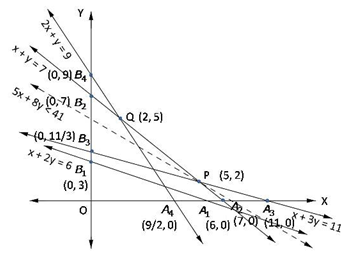Unbounded shaded region A2 P Q B4 represents feasible region with corner points A3(11, 0), P(5, 2), Q(2, 5), B4(0, 9)

The value of Z = 5x + 8y at

A3(11, 0) = 5(11) + 8(0) = 55

P(5, 2) = 5(5) + 8(2) = 41

Q(2, 5) = 5(2) + 8(5) = 50

B4(0, 9) = 5(0) + 8(9) = 72

Hence, smallest value of Z = 41

Open half plane 5x + 8y < 41 has no point in common with feasible region

So, smallest value is the minimum value.

Last cost of mixture = Rs 41